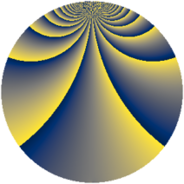# Properties

 Label 345.2.xLevel $345$ Weight $2$ Character orbit 345.x Rep. character $\chi_{345}(2,\cdot)$ Character field $\Q(\zeta_{44})$ Dimension $880$ Newform subspaces $1$ Sturm bound $96$ Trace bound $0$

# Related objects

## Defining parameters

 Level: $$N$$ $$=$$ $$345 = 3 \cdot 5 \cdot 23$$ Weight: $$k$$ $$=$$ $$2$$ Character orbit: $$[\chi]$$ $$=$$ 345.x (of order $$44$$ and degree $$20$$) Character conductor: $$\operatorname{cond}(\chi)$$ $$=$$ $$345$$ Character field: $$\Q(\zeta_{44})$$ Newform subspaces: $$1$$ Sturm bound: $$96$$ Trace bound: $$0$$

## Dimensions

The following table gives the dimensions of various subspaces of $$M_{2}(345, [\chi])$$.

Total New Old
Modular forms 1040 1040 0
Cusp forms 880 880 0
Eisenstein series 160 160 0

## Trace form

 $$880q - 22q^{3} - 44q^{6} - 36q^{7} + O(q^{10})$$ $$880q - 22q^{3} - 44q^{6} - 36q^{7} - 36q^{10} - 22q^{12} - 28q^{13} - 34q^{15} - 82q^{18} - 52q^{21} - 112q^{22} - 44q^{25} - 34q^{27} - 20q^{28} - 38q^{30} - 72q^{31} - 48q^{33} - 44q^{36} - 84q^{37} - 108q^{40} + 10q^{42} - 12q^{43} - 20q^{45} - 88q^{46} - 94q^{48} - 20q^{51} - 296q^{52} - 20q^{55} + 18q^{57} - 52q^{58} - 42q^{60} - 16q^{61} - 90q^{63} + 60q^{66} - 60q^{67} + 16q^{70} - 58q^{72} - 68q^{73} - 74q^{75} + 72q^{76} + 78q^{78} + 52q^{81} - 60q^{82} - 76q^{85} + 18q^{87} + 12q^{88} - 436q^{90} - 80q^{91} + 12q^{93} + 212q^{96} + 96q^{97} + O(q^{100})$$

## Decomposition of $$S_{2}^{\mathrm{new}}(345, [\chi])$$ into newform subspaces

Label Dim. $$A$$ Field CM Traces $q$-expansion
$$a_2$$ $$a_3$$ $$a_5$$ $$a_7$$
345.2.x.a $$880$$ $$2.755$$ None $$0$$ $$-22$$ $$0$$ $$-36$$